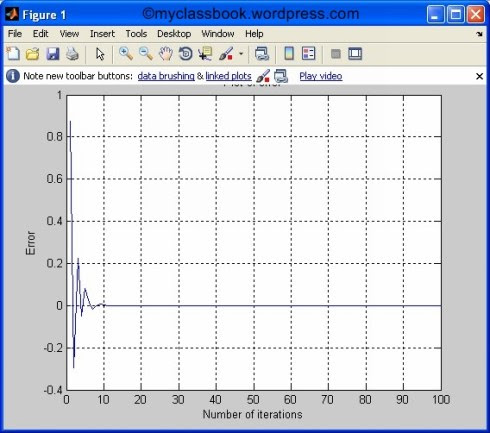Bisection Method Matlab Program With Flowchart & Algorithm

There are various methods available for finding the roots of given equation such as Bisection method, False position method, Newton-Raphson method, etc. Today I am going to explain Bisection method for finding the roots of given equation. I will also explain MATLAB program for Bisection method.

Bisection method is very simple but time-consuming method. In this method, we first define an interval in which our solution of the equation lies. As the name indicates, Bisection method uses the bisecting (divide the range by 2) principle. In this method, we minimize the range of solution by dividing it by integer 2.

Following are the steps to find the approximate solution of given equation using Bisection method:

Let us assume that we have to find out the roots of f(x), whose solution lies in the range (a,b), which we have to determine. The only condition for bisection method is that f(a) and f(b) should have opposite signs (f(a) negative and f(b) positive). When f(a) and f(b) are of opposite signs at least one real root between ‘a’ and ‘b’ should exist.

For the first approximation, we assume that root to be,

x0=(a+b)/2

Then we have to find a sign of f(x0).
If f(x0) is negative the root lies between a and x0. If f(x0) is positive the root lies between x0 and b.
Now we have new minimized range, in which our root lies.
The next approximation is given by,

• x1 = (a+x0)/2………….if f(x0) is negative

• x1 = (x0+b)/2………….if f(x0) is positive

In this taking midpoint of the range of approximate roots, finally, both values of range converge to a single value, which we can take as an approximate root.

Bisection Method Example:

Find the root of x^3 - x = 1 by using Bisection Method.

Solution:

Let us assume that the root of x^3 - x - 1=0 lies between (1,2)

Here, f(1) = negative and f(2) = positive.

Hence root lies between (1,2)

For first approximation,

x0 = (1+2)/2 = 1.5
f(x0) = f(1.5) = positive
Hence root lies between (1,1.5)

x1 = (1+1.5)/2 = 1.25
f(x1) = f(1.25) = negative
Hence root lies between (1.25,1.5)

x2 = (1.25+1.5)/2 = 1.375
f(x2) = f(1.375) = positive
Hence root lies between (1.25,1.375)

x3 = (1.25+1.375)/2 = 1.3125
f(x3) = f(1.3125) = negative
Hence root lies between (1.3125,1.375)

x4 = (1.3125+1.375)/2 = 1.34375
f(x4) = f(1.34375) = positive
Hence root lies between (1.3125,1.34375)

x5 = (1.3125+1.34375)/2 = 1.328125
f(x5) = f(1.328125) = positive
Hence root lies between (1.3125,1.328125)

x6 = (1.3125+1.328125)/2 = 1.320313
f(x6) = f(1.320313) = negative
Hence root lies between (1.320313,1.328125)

x7 = (1.320313+1.328125)/2 = 1.324219
f(x7) = f(1.324219) = negative
Hence root lies between (1.324219,1.328125)

x8 = (1.324219+1.328125)/2 = 1.326172
f(x8) = f(1.326172) = positive
Hence root lies between (1.324219,1.326172)

x9 = (1.324219+1.326172)/2 = 1.325195
f(x9) = f(1.325195) = positive
Hence root lies between (1.324219,1.325195)

x10 = (1.324219+1.325195)/2 = 1.324707
f(x10) = f(1.324707) = negative
Hence root lies between (1.324707,1.325195)

x11 = (1.324707+1.325195)/2 = 1.324951
f(x11) = f(1.324951) = positive
Hence root lies between (1.324707,1.324951)

x12 = (1.324707+1.324951)/2 = 1.324829
f(x12) = f(1.324829) = positive
Hence root lies between (1.324707,1.324829)

x13 = (1.324707+1.324829)/2 = 1.324768
f(x13) = f(1.324768) = positive
Hence root lies between (1.324707,1.324768)

OK stop it …. I know you are getting tired… but there is no shortcut.

Now if you observe two limits (1.324707, 1.324768) of above range, they are almost same, which is our root of given equation.

Algorithm for Bisection Method:

1. Input function and limits.

2. Repeat steps 3 and 4 100 times.

3. x=(a+b)/2

4. If f(x0)<0, a=x  else b=x

5. Display x

6. Repeat steps 7 and 8 10 times.

7. Error = x-(a+b)/2

8. Store error values in array

9. Plot error

10. STOP.

I would recommend watching below video for complete understanding for Bisection method algorithm.

MATLAB program for bisection method:

% Crated by mayclassbook.org auther: mayuresh
% Created on 12 june 2013
% Bisection method
% Find the roots of x^3-x-1 using bisection method and plot the error
f=@(x) x^3-x-1;
a=1;
b=2;
for i=1:100
c=(a+b)/2;
if f(c)>0
b=c;
else a=c;
end
end
a=1; b=2; p=c;
for i=1:100
c=(a+b)/2;
er(i)=f(c)-f(p);
if f(c)>0
b=c;
else a=c;
end
end
fprintf('Root of given equation is %f',c)
plot(er);
title('Plot of error')
xlabel('Number of iterations')
ylabel('Error')
grid on;

The plot for error between interval is given by,I would recommend watching below video for complete understanding for Bisection method example

You may also like

1.2.3.